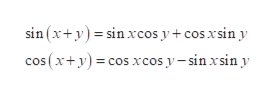# Find the exact values of x and y of the following: -400 degrees -150 degrees 250 degrees  do not enter cos or sin, use exact values

Question

Find the exact values of x and y of the following:

-400 degrees

-150 degrees

250 degrees

do not enter cos or sin, use exact values

check_circleExpert Solution
Step 1

The period of a function is defined as the interval where the function repeats itself.

Step 2

The period of sin and cos is 360°.

So get the values of sin (-400°) and cos (-400°).

Step 3

The expansion formula is to b...help_outlineImage Transcriptionclosesin (x+y)sin xcos y + cos xsin y cos (x+y)cos xcos y-sin xsin y fullscreen

### Want to see the full answer?

See Solution

#### Want to see this answer and more?

Solutions are written by subject experts who are available 24/7. Questions are typically answered within 1 hour*

See Solution
*Response times may vary by subject and question
Tagged in

### Other